Engineering ToolBox - Resources, Tools and Basic Information for Engineering and Design of Technical Applications!

# Center of Gravity

## A body and the center of gravity.

The "center of gravity" for a volume, an area or a line - is the point at which the body - if suspended - would be in balance.

For a symmetrical body in a uniform material - the center of gravity would be in the geometric center.

### Perimeter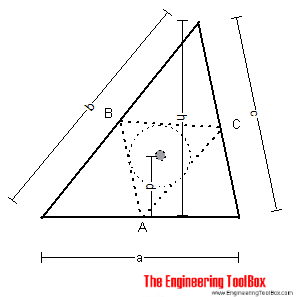The center of gravity is the center of the circle inscribed in triangle ABC (middle points of the sides of the triangle).

The distance d can be calculated as

d = h (b + c) / 2 (a + b + c)                                (1)

### Triangle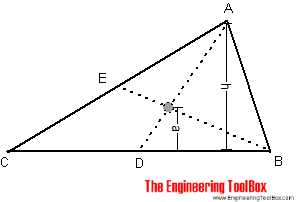The center of gravity of a triangle is at the intersection of lines BE and AD. The distance a can be calculated as

a = h / 3                               (2)

### Parallelogram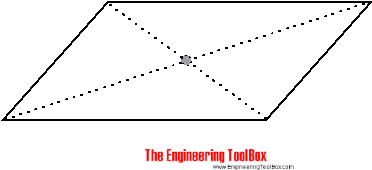The center of gravity of a parallelogram is at the intersection of the diagonals.

### Trapezoid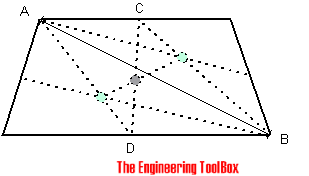The center of gravity of a trapezoid can be estimated by dividing the trapezoid in two triangles. The center of gravity will be in the intersection between the middle line CD and the line between the triangles centers of gravity.

### Two Bodies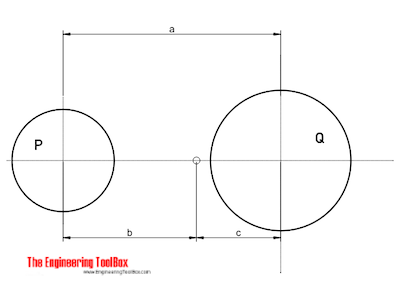The center of gravity of two bodies can be calculated as

b = Q a / (P + Q)                     (3a)

c = P a / (P + Q)                     (3b)

where

Q, P = weight or mass of the bodies (N, kg, lb, slugs)

## Related Topics

• ### Mathematics

Mathematical rules and laws - numbers, areas, volumes, exponents, trigonometric functions and more.
• ### Mechanics

Forces, acceleration, displacement, vectors, motion, momentum, energy of objects and more.
• ### Miscellaneous

Engineering related topics like Beaufort Wind Scale, CE-marking, drawing standards and more.
• ### Statics

Loads - forces and torque, beams and columns.

## Related Documents

• ### Acceleration of Gravity and Newton's Second Law

Acceleration of gravity and Newton's Second Law - SI and Imperial units.
• ### Area Moment of Inertia - Typical Cross Sections I

Typical cross sections and their Area Moment of Inertia.
• ### Buoyancy

Buoyancy is the resultant force acting on a submerged body.
• ### Center Mass

Calculate position of center mass.
• ### Center of Gravity and Buoyancy

Stability - the center of gravity vs. the center of buoyancy.
• ### Force

Newton's third law - force vs. mass and acceleration.
• ### Hydrostatic Force acting on Submerged Surface

Calculate the thrust force acting on a submerged surface.
• ### Mass vs. Weight

Mass vs. weight - the Gravity Force.
• ### Pythagorean Theorem

Verifying square corners.
• ### Regular Polygons

Areas of regular polygons - polygons with 3 to 12 sides.
• ### Right Angled Triangle

Right angled triangle equations.
• ### Section Modulus - Unit Converter

Convert between Elastic Section Modulus units.
• ### Triangle

Triangle analytical geometry.
• ### Universal Gravitational Law

Gravitational attraction between two objects vs. mass of the objects and the distance between them.

## Engineering ToolBox - SketchUp Extension - Online 3D modeling!

Add standard and customized parametric components - like flange beams, lumbers, piping, stairs and more - to your Sketchup model with the Engineering ToolBox - SketchUp Extension - enabled for use with older versions of the amazing SketchUp Make and the newer "up to date" SketchUp Pro . Add the Engineering ToolBox extension to your SketchUp Make/Pro from the Extension Warehouse !

We don't collect information from our users. More about

## Citation

• The Engineering ToolBox (2008). Center of Gravity. [online] Available at: https://www.engineeringtoolbox.com/center-gravity-d_1310.html [Accessed Day Month Year].

Modify the access date according your visit.

9.19.12